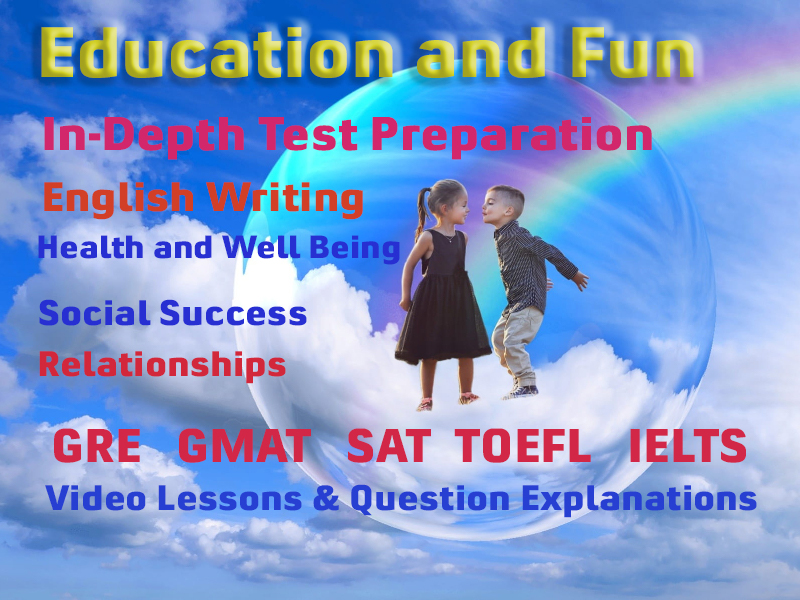# ISSB Coding Decoding Questions

Giving code of Artificial values to Letter of alphabet. The word code means - a collection or digit of law, a system of rules and regulation, established principles or standards, a system of words, letters or symbols which represent sentences or other words, to ensure economy or secrecy in transmission. In the service of army, perfect service is required about the information with regard to the movement or actions of the army .For this object often secret messages are to be conveyed in code and the same have to be decoded. Hence it is essential that the candidates should be in a position to trace the key to the code.

Letters of alphabet in this test do not stand for themselves but they stand for some other letters, i.e., they keep an artificial or code values. These artificial or code values are given to these alphabets according to some fixed law or pattern which are not difficult for the candidate to understand. By following the same law or principle the coded message can be decoded in to clear word message. This test can be solved with convenience, if all the letters of the alphabet are first written and then, by compositing the real and artificial value of letter, set the relationship or pattern of the code .For this object, usually, alphabets are printed on the answer sheet for the use of the candidate.

In this kind of intelligence test, the digits do not carry their real values, but they are given artificial values. These artificial values are given tot eh digits according to fixed principles which can be very easily understood by a minute observation.

### Example:

• If 2 = 0 And 3 = 3 And 4 = 8 Then 7 = ?
• A. 30
• B. 35
• C. 40

Try to find out the underlying factors to solve this coding value question.

Solution: In this question number (or figure) 2 is multiplied by zero, and, therefore, it is equivalent to zero. Number 3 is multiplied by 1, and therefore, it is equivalent to 3. Figure or number 4 is multiplied by 2, and, therefore, it is equivalent to 8. Number 5 is multiplied by 3, and, therefore, it is equivalent to 15. Careful observation will disclose that the number by which each succeeding figure is multiplied goes on increasing by number one, in order to assume an artificial value. Using the same principle, the figure 7 is to be multiplied by 5 Therefore, its artificial or coded value.

Therefore, its artificial or coded value will be 7 X 5 = 35.

But note that the answer to the question will be choice (2) and not number or figure 35. Hence the answer is choice (2). Answer Choice (2)

### Underlying Factors

The underlying factors are normally based on simple arithmetic i.e. addition, subtraction, multiplication, and division.

### Example

• If Y C V G T means W A T E R then what does H K T G means.

Solution: In this question

• Y stands for W
• C stand for A
• V stand for T
• G stand for E
• T stand for R

A careful observation brings out that each letter in the alphabet ( A B C D E F G H I J K L M N O P Q R S T U V W X Y Z) stands for the letter two paces preceding it .Using the same principle ,the letters HKTG will stand for FIRE. It may be noted that in the ISSB tests ,the method of giving the same question is usually ,as under .Which choice mentions the first letter of the correct answer to the following .If Y C V G T means W A T E R ,what does H K G T means .Choices. (i) F , (ii) R, (iii) E. The answer is obviously (i) as F is the first letter of the correct word which is FIRE .The candidate will be required to write figure [i] in the suitable column in the answer sheet provided .The candidates should not write the word FIRE, because then, the answer will be not. Giving Artificial or Code Values to Arithmetical Digits.

In this kind of intelligence test, the digits do not carry their real values, but they are given artificial values .These artificial values are given to the digits according to fixed principles which can be very easily understood by a minute observation.

### More Educational and Fun StuffMore In-depth knowledge about what you need. Detailed about test preparation, English Writing, TOEFL, and IELTS.